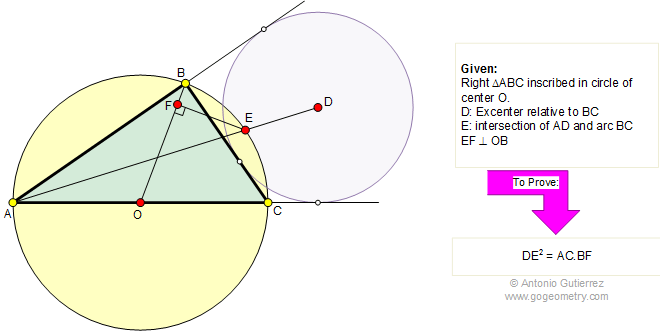Problem 294: Right triangle, Circumcenter, Excenter, Hypotenuse, Perpendicular. Level: High School, College, SAT Prep.

The figure shows a right triangle ABC inscribed in a circle of center O (circumcenter). D is the excenter relative to BC and E is the point of intersection of AD and arc BC. If EF is perpendicular to OB, prove that DE2 = AC.BF.Geometry problem solving is one of the most challenging skills for students to learn. When a problem requires auxiliary construction, the difficulty of the problem increases drastically, perhaps because deciding which construction to make is an ill-structured problem. By “construction,” we mean adding geometric figures (points, lines, planes) to a problem figure that wasn’t mentioned as "given."